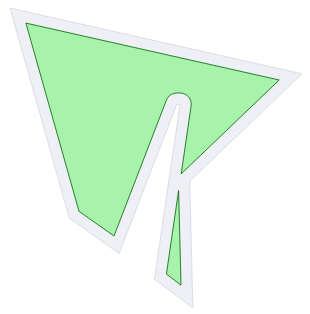# ClipperOffset.Execute

Del.» procedure Execute(out solution: TPaths; Delta: Double); overload;

C++ » void Execute(Paths& solution, double delta);

C#  » public void Execute(ref Paths solution, double delta);

Del.» procedure Execute(out PolyTree: TPolyTree; Delta: Double); overload;

C++ » void Execute(PolyTree& polytree, double delta);

C#  » public void Execute(ref PolyTree polytree, double delta);

This method takes two parameters. The first is the structure (either PolyTree or Paths) that will receive the result of the offset operation. The second parameter is the amount to which the supplied paths will be offset - negative delta values to shrink polygons and positive delta to expand them.

This method can be called multiple times, offsetting the same paths by different amounts (ie using different deltas).```#include "clipper.hpp" ... using namespace ClipperLib; int main() { Path subj; Paths solution; subj << IntPoint(348,257) << IntPoint(364,148) << IntPoint(362,148) << IntPoint(326,241) << IntPoint(295,219) << IntPoint(258,88) << IntPoint(440,129) << IntPoint(370,196) << IntPoint(372,275); ClipperOffset co; co.AddPath(subj, jtRound, etClosedPolygon); co.Execute(solution, -7.0); //draw solution ... DrawPolygons(solution, 0x4000FF00, 0xFF009900); } ```